RRB JE ECE (CBT I) Mock Test- 4

# RRB JE ECE (CBT I) Mock Test- 4

Test Description

## 100 Questions MCQ Test RRB JE Mock Test Series for ECE | RRB JE ECE (CBT I) Mock Test- 4

RRB JE ECE (CBT I) Mock Test- 4 for Railways 2022 is part of RRB JE Mock Test Series for ECE preparation. The RRB JE ECE (CBT I) Mock Test- 4 questions and answers have been prepared according to the Railways exam syllabus.The RRB JE ECE (CBT I) Mock Test- 4 MCQs are made for Railways 2022 Exam. Find important definitions, questions, notes, meanings, examples, exercises, MCQs and online tests for RRB JE ECE (CBT I) Mock Test- 4 below.
Solutions of RRB JE ECE (CBT I) Mock Test- 4 questions in English are available as part of our RRB JE Mock Test Series for ECE for Railways & RRB JE ECE (CBT I) Mock Test- 4 solutions in Hindi for RRB JE Mock Test Series for ECE course. Download more important topics, notes, lectures and mock test series for Railways Exam by signing up for free. Attempt RRB JE ECE (CBT I) Mock Test- 4 | 100 questions in 90 minutes | Mock test for Railways preparation | Free important questions MCQ to study RRB JE Mock Test Series for ECE for Railways Exam | Download free PDF with solutions
 1 Crore+ students have signed up on EduRev. Have you?
RRB JE ECE (CBT I) Mock Test- 4 - Question 1

### If sinθ + sin2θ = 1, then the value of cos12θ + 3 cos10θ + 3 cos8θ + cos6θ – 1 is:

Detailed Solution for RRB JE ECE (CBT I) Mock Test- 4 - Question 1 sinθ + sin2θ = 1 ⇒ sinθ = cos2θ

Given : cos12θ + 3 cos10θ + 3 cos8θ + cos6θ–1

= (cos4θ + cos2θ)3 – 1 = (sin2θ + cos2θ)3 – 1 = 1 – 1 = 0

RRB JE ECE (CBT I) Mock Test- 4 - Question 2

### The height of an equilateral triangle is 4√3 cm, then what will be the area of the equilateral triangle?

Detailed Solution for RRB JE ECE (CBT I) Mock Test- 4 - Question 2

Height = 4 cm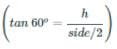Area of equilateral triangle = √3/4 x 82 = 16√3 cm2.

RRB JE ECE (CBT I) Mock Test- 4 - Question 3

### If 10 men can do work in 6 days and 15 women can do the same in 5 days, Then 8 men and 5 women can together do the work in :

Detailed Solution for RRB JE ECE (CBT I) Mock Test- 4 - Question 3 10 Men can do the work in 6 days

If the work is to be finished in 5 days, number of women required = 15

If the work is to be finished in 6 days, number of women required =[15×5]/6 = 25/2

So that 10 men = 25/2 women

or 5 women = 4 men

8 men + 5 women = 8 men + 4 men = 12 men

Now 10 men can finish the work in = 6 days

∴ 12 men can finish the work in [6×10]/12=5 days

RRB JE ECE (CBT I) Mock Test- 4 - Question 4

The average of two numbers is 8, and that of another three numbers is 3. The average of these five numbers is-

Detailed Solution for RRB JE ECE (CBT I) Mock Test- 4 - Question 4 Sum of two numbers = 8 ×2 = 16

Sum of other three numbers = 3 × 3 = 9

Total sum = 25

Average = 25/5 = 5

RRB JE ECE (CBT I) Mock Test- 4 - Question 5

Which of the following is obtained after the rationalization of the expression 1/(√2+√3+√5) ?

Detailed Solution for RRB JE ECE (CBT I) Mock Test- 4 - Question 5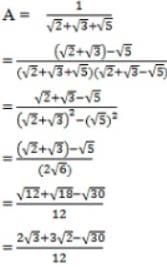RRB JE ECE (CBT I) Mock Test- 4 - Question 6

If sin θ=8/17, where 0o<θ<90o, then tan θ+sec θ is:

Detailed Solution for RRB JE ECE (CBT I) Mock Test- 4 - Question 6 Using pythagoras theorem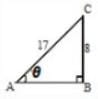AC2 = AB2 + BC2

(17)2 = AB2 + (8)2

AB2 = 289 - 64

AB = √225

AB = 15

∴ tan θ = 8/15 and sec θ = 17/15

∴ tan θ + sec θ = 8/15 + 17/15 = 25/15 = 5/3

RRB JE ECE (CBT I) Mock Test- 4 - Question 7

OA, OB, OC are 3 lines in a plane which meet at O. If the angles ∠AOB, ∠BOC, ∠COA are 2x, 5x, 8x respectively (where x is a positive angle), then x equals:

Detailed Solution for RRB JE ECE (CBT I) Mock Test- 4 - Question 7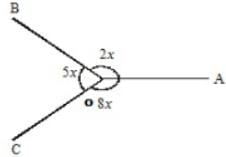2x + 5x + 8x = 360°

15x + 360°

x = 360°/15 = 24°

RRB JE ECE (CBT I) Mock Test- 4 - Question 8

The value of tan 31° tan 33° ... tan 59° is equal to:

Detailed Solution for RRB JE ECE (CBT I) Mock Test- 4 - Question 8

tan 31°. tan 32°. tan 33° .... tan 59°

= tan 31°. tan 32°. tan 33° ... tan 57°. tan 58°. tan 59°

= tan 31º. tan 32°. tan 33° ... cot 33°. cot 32°. cot 31° = 1

[tan (90°-θ) = cotθ and tanθ cotθ =1]

RRB JE ECE (CBT I) Mock Test- 4 - Question 9

If x = 81, then the value of (x1/4 -1) (x1/4+1) is:

Detailed Solution for RRB JE ECE (CBT I) Mock Test- 4 - Question 9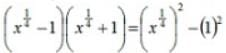x1/2 - 1 = (81)1/2 - 1 = 9 - 1 = 8

RRB JE ECE (CBT I) Mock Test- 4 - Question 10

A man sells two scooters for Rs.12000 each. He makes a profit of 20% on one and a loss of 20% on the other. The profit/loss, on the whole, is

Detailed Solution for RRB JE ECE (CBT I) Mock Test- 4 - Question 10

SP1 = 12000

profit = 20%

∴ CP1 = 12000/120 x 100 = Rs.10,000

SP2 = 12000

Loss % = 20%

∴ CP = 12000/80 x 100 = Rs.15,000

Total CP = 25000

Total SP = 24000

Loss = Rs.25000 – Rs.24000 = Rs.1000

(or)

If the selling price of the two items is equal and the percentage of profit on one equals the percentage of loss on the other, then, the result is always loss and the percentage of loss is (loss%2/100).

Hence, in the present case, loss percentage = (20)2/100 percent = 4%.

Loss = 4/96 × Rs.24000 = Rs.1000

RRB JE ECE (CBT I) Mock Test- 4 - Question 11

A train covers a certain distance in 50 minutes, if it runs at a speed of 48 km/hr on an average. The speed at which the train must run so that the time of journey becomes 40 minutes?

Detailed Solution for RRB JE ECE (CBT I) Mock Test- 4 - Question 11 We are having time and speed given, so first we will calculate the distance. Then we can get a new speed for a given time and distance.

Time = 50/60 hr = 5/6 hr

Speed = 48 mph

Distance = S*T = 48 * 5/6 = 40 km

New time will be 40 minutes so,

Time = 40/60 hr = 2/3 hr

Now we know,

Speed = Distance/Time

New speed = 40*3/2 kmph = 60kmph

RRB JE ECE (CBT I) Mock Test- 4 - Question 12

The pie chart given here shows expenditures incurred by a family on various items and their savings, which amount to Rs. 8,000 in a month.

Study the chart and answer the questions based on the pie chart.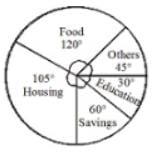How much expenditure is incurred on education?

Detailed Solution for RRB JE ECE (CBT I) Mock Test- 4 - Question 12

∴ 60° = Rs8000

∴ Total expenditure

300° = 8000/60 x 300 = rs 40,000

Expenditure on education

= 30/300 x 40,000 = rs 4000

RRB JE ECE (CBT I) Mock Test- 4 - Question 13

The pie chart given here shows expenditures incurred by a family on various items and their savings, which amount to Rs. 8,000 in a month.

Study the chart and answer the questions based on the pie chart.The ratio of the expenditure on food to the savings is:

Detailed Solution for RRB JE ECE (CBT I) Mock Test- 4 - Question 13 Expenditure on food

=(120/300)×40,000=₹16,000

Required ratio = 16000 : 8000 ⇒ 2 : 1

RRB JE ECE (CBT I) Mock Test- 4 - Question 14

A solid metallic cone of height 10 cm and radius of base 20 cm is melted to make spherical balls each of 4 cm diameter. How many such balls can be made?

Detailed Solution for RRB JE ECE (CBT I) Mock Test- 4 - Question 14 Volume of cone = 1/3πr2h

= (1/3)×π×(20)2×10

= 400×10

= (π/3)×4000=(4000/3)π

Volume of spherical balls = 4/3πr3

= (4/3)×π×(2)2

= 32/3π

∴ Spherical balls are formed from the cones.

∴ Total volume of all spherical cones = Volume of cones.

∴ No. of spherical balls = Volume of cone/Vol of 1 spherical ball

= 4000/3

= 32/3

= 4000/32=125

RRB JE ECE (CBT I) Mock Test- 4 - Question 15

Sum of the lengths of any two sides of a triangle is always greater than

Detailed Solution for RRB JE ECE (CBT I) Mock Test- 4 - Question 15 The sum of the other two sides of a triangle is always greater than the third side.
RRB JE ECE (CBT I) Mock Test- 4 - Question 16

If D is a point on the side AB of triangle ABC and DE is a line through D meeting AC at E such that ∠ADE = ∠ACB, then AB. AD is equal to:

Detailed Solution for RRB JE ECE (CBT I) Mock Test- 4 - Question 16 Clearly ΔADE and ΔABC are similar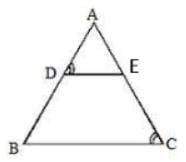∴ AD/AC = AE/AB

AD. AB = AE. AC

RRB JE ECE (CBT I) Mock Test- 4 - Question 17

A man can row 15 km per hour downstream and 9 km per hour upstream. The speed (in km/hour) of the boat in still water is:

Detailed Solution for RRB JE ECE (CBT I) Mock Test- 4 - Question 17

The Speed of Boat in still water = 1/2 (Rate downstream + Rate upstream)

The speed of the boat in still water = 1/2 (15 +9) = 24/2 = 12 km/hr.

RRB JE ECE (CBT I) Mock Test- 4 - Question 18

If x : y = 3 : 4, then the value of (5x - 2y)/(7x+2y)

Detailed Solution for RRB JE ECE (CBT I) Mock Test- 4 - Question 18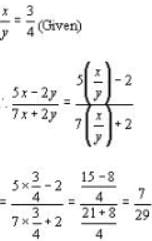RRB JE ECE (CBT I) Mock Test- 4 - Question 19

The sum of the numerator and denominator of a certain fraction is 8. If 2 is added to both the numerator and the denominator the value of the fraction increased by 4/35, then the fraction is:

Detailed Solution for RRB JE ECE (CBT I) Mock Test- 4 - Question 19

x + y = 8 ……(i)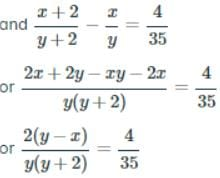or 35 (y - x) = 2y (y + 2) (ii)

from (i)

x = 8 - y

substituting this value in (ii)

35 (y - 8 + y) - 2y (y + 2)

35 (y - 4) = y (y + 2)

y2 - 33y + 140 = 0

y = 28 or 5

We can't take 28, taking y = 5, x will become 3

Therefore x/y = 3/5

RRB JE ECE (CBT I) Mock Test- 4 - Question 20

The simple interest on a certain principal at the rate of 9.5 percent per annum is Rs 950 for two year. How much will be the additional interest on the same amount for the same period at the rate of 10.5 percent per annum?

Detailed Solution for RRB JE ECE (CBT I) Mock Test- 4 - Question 20

S.I. = Rs 950, rate = 9.5%

time = 2 years

∴ sum = (950×100)/(9.5×2)=5000

If rate of interest is 10.5% then

Simple Interest = (5000×2×10.5)/100 = Rs 1050

∴ additional interest due to change in rate of interest = Rs 100

RRB JE ECE (CBT I) Mock Test- 4 - Question 21

If the angle of elevation of the top of a tower from two points at the distance x and y meter from the base and in the same straight line with it are complementary, then the height of the tower is?

Detailed Solution for RRB JE ECE (CBT I) Mock Test- 4 - Question 21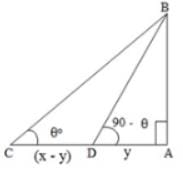Let AB be the tower and C and D be the point of observation on AC.

∠AC B = θ, ∠ADB = 90 - θ, AB = h m

AC = X m, AD = Y m

So, CD = x - y m.

tan θ = h/x

And tan (90 - θ) = h/y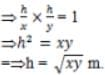RRB JE ECE (CBT I) Mock Test- 4 - Question 22

Kamya purchased an item of 46,000 and sold it at a loss of 12%. With that amount she purchased another item and sold it at a gain of 12%. What was her overall gain/loss?

Detailed Solution for RRB JE ECE (CBT I) Mock Test- 4 - Question 22 SP of first article = (46000×88)100=₹40,480

SP for second article = (40480×112)/100=₹45,337.60

∴ Loss = 46000 - 45337.60 = ₹662.40.

RRB JE ECE (CBT I) Mock Test- 4 - Question 23

The owner of a cell phone shop charges his customer 23% more than the cost price. If a customer paid Rs.7,011 for a cell phone, then what was the cost price of the cell phone?

Detailed Solution for RRB JE ECE (CBT I) Mock Test- 4 - Question 23 Let the cost price of cell phone be Rs. x

According to the question,

[(100+23)/100] × x = 7011

Or, x = (7011×100)/123 = 5700

RRB JE ECE (CBT I) Mock Test- 4 - Question 24

The cost of an article was Rs.75. The cost was first increased by 20% and later on it was reduced by 20%. The present cost of the article is:

Detailed Solution for RRB JE ECE (CBT I) Mock Test- 4 - Question 24 Effective decrease

= [20 – 20 – 20×(20/100)] % = -4%

∴ Present cost of the article = 96% of Rs.75

= (75×96)/100 = Rs.72

RRB JE ECE (CBT I) Mock Test- 4 - Question 25

A rectangular box measures internally 1.6 m long, 1 m broad and 60 cm deep. The number of cubical block each of edge 20 cm that can be packed inside the box is:

Detailed Solution for RRB JE ECE (CBT I) Mock Test- 4 - Question 25 Volume of rectangular box = 160 x 100 x 60 cm3

and volume of one cubical block = 20 x 20 x 20 cm3

∴ Required number of cubical block = (160×100×60)/(20×20×20) = 120 blocks

RRB JE ECE (CBT I) Mock Test- 4 - Question 26

The diameter of the curved surface of a bucket is 28 decimeter and 14 decimeters and its height is 12 decimeter. Find its volume.

Detailed Solution for RRB JE ECE (CBT I) Mock Test- 4 - Question 26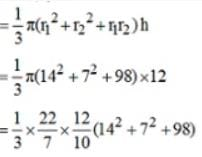RRB JE ECE (CBT I) Mock Test- 4 - Question 27

What will be the area of a rhombus, whose one side is 20 cm and one diagonal is 24 cm?

Detailed Solution for RRB JE ECE (CBT I) Mock Test- 4 - Question 27 Let length of another diagonal = 2x

Diagonals of rhombus intersect at right angles, so by using pythagoras theorem,

x2 + 122 = 202

Therefore x = 16 , So diagonal = 2'16 =32

Area of rhombus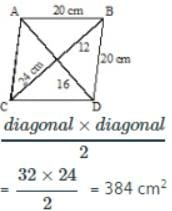RRB JE ECE (CBT I) Mock Test- 4 - Question 28

The ratio of two unequal sides of a rectangle is 1 : 2. If its perimeter is 24 cm, then the length of diagonal in cm is:

Detailed Solution for RRB JE ECE (CBT I) Mock Test- 4 - Question 28 Let the sides of rectangle be 2x, x cm

∴ Perimeter of rectangle = 2 (2x+x)

2 (2x+x) = 24

or 6x = 24

∴ x = 4 cm

hence length of diagonal = √[(2x)2+x2]

= √5x2

= √(5×16)

=√ 80

= 4√5

RRB JE ECE (CBT I) Mock Test- 4 - Question 29

8 hours of work for a woman is equal to 6 hours of work for a man or 12 hours of work for a boy. If 9 men working 6 hours daily can complete a work in 6 days, then in how many days 12 men, 12 women and 12 boys working 8 hours daily will complete the same work?

Detailed Solution for RRB JE ECE (CBT I) Mock Test- 4 - Question 29 1 man done work in 6×6×9 hours

Since 8W=6M=12B

therefore 12M+12W+12B=27M

therefore 27 men will do work in (6×6×9/27) = 12 hours

= 12/8=1.5 days

RRB JE ECE (CBT I) Mock Test- 4 - Question 30

In an examination, the average marks was found to be 50. For deducting marks for computational errors, the marks of 100 candidates had to be changed from 90 to 60 each and so the average of marks came down to 45. The total number of candidates, who appeared at the examination, was:

Detailed Solution for RRB JE ECE (CBT I) Mock Test- 4 - Question 30 Let the total number of candidates be x.

∴ 50x - 30 × 100 = 45x

⇒ 5x = 3000

⇒ x = 3000/5 = 600

RRB JE ECE (CBT I) Mock Test- 4 - Question 31

The series is given, with one term missing. Choose amongst the given responses and complete the series.

ABZ, CDY, EFX, GHW, __.

Detailed Solution for RRB JE ECE (CBT I) Mock Test- 4 - Question 31 A and B are first and second letters respectively as per alphabetical order.

Z is the last letter as per alphabetical order.

C and D are 3rd and 4th letter respectively as per alphabetical order.

Y is the 2nd last letter as per alphabetical order.

Following the similar pattern, we get IJV.

RRB JE ECE (CBT I) Mock Test- 4 - Question 32

Each of the questions below consists of a question and two statements numbered I and II given below it. You have to decide whether the data provided in which of the statements are sufficient to answer the question. Choose your answer from the options based on this.

What can be the code for '12'?

I. If 'For your sake' is written as '13 12 21'.

II. If 'Don't leave for tomorrow' is written as '31 13 14 11'.

Detailed Solution for RRB JE ECE (CBT I) Mock Test- 4 - Question 32 Neither of the statements alone is sufficient to answer and even if we combine the two statements, we get that 13 = for. But, 12 = '?'. Hence, the answer is (4).
RRB JE ECE (CBT I) Mock Test- 4 - Question 33

If ANIMAL is coded as 321435 and ELEPHANT is coded as 65609328, then METAL is coded as

Detailed Solution for RRB JE ECE (CBT I) Mock Test- 4 - Question 33 As per the codes given in the question,

M is coded as 4, E is coded as 6, T is coded as 8, A is coded as 3, L is coded as 5.

RRB JE ECE (CBT I) Mock Test- 4 - Question 34

T is to the north-east of D and east of S. How is D related to H, if H is to the east of T?

Detailed Solution for RRB JE ECE (CBT I) Mock Test- 4 - Question 34

D is south-west of H.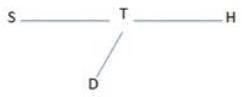RRB JE ECE (CBT I) Mock Test- 4 - Question 35

In the following question, which one set of letters when sequentially placed at the gaps in the given letter series shall complete it?

a _ b d _ b _ c a b _ _ a b b _

Detailed Solution for RRB JE ECE (CBT I) Mock Test- 4 - Question 35

a b b d/ a b b c/ a b b d/ a b b c

RRB JE ECE (CBT I) Mock Test- 4 - Question 36

Which of the following will be the mirror image of the given question figure (x), if the mirror is placed along the line MN. Choose the correct mirror image among (I), (II), (III) and (IV) given.

Question Figure: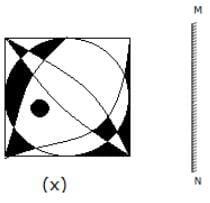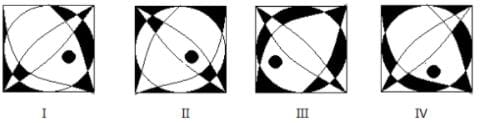Detailed Solution for RRB JE ECE (CBT I) Mock Test- 4 - Question 36

Image I is the correct mirror image of (x).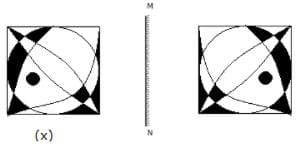RRB JE ECE (CBT I) Mock Test- 4 - Question 37

Arrange the given words in a meaningful sequence.

1. Kilogram

2. Gram

3. Ton

4. Quintal

Detailed Solution for RRB JE ECE (CBT I) Mock Test- 4 - Question 37

With respect to the values -

Ton (1000 kgs) > Quintal (100 Kgs) > Kilogram (1000 grams) > Gram

RRB JE ECE (CBT I) Mock Test- 4 - Question 38

Pointing to a photograph, Prakash said "Her father's only son is the father of my brother". How is that person in the photograph related to Prakash's father?

Detailed Solution for RRB JE ECE (CBT I) Mock Test- 4 - Question 38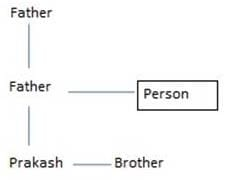The person in the photograph is the sister of Prakash’s Mother

RRB JE ECE (CBT I) Mock Test- 4 - Question 39

In a certain code language, " play your good game" is coded as " ad pd cd md" , "play game run fast" is coded as " zd cd md td" , "run speed end game" is coded as " fd zd ld md". Find out what will be the code for " your good"?

Detailed Solution for RRB JE ECE (CBT I) Mock Test- 4 - Question 39 " play your good game" is coded as " ad pd cd md"..(I)

"play game run fast" is coded as " zd cd md td"..(II)

"run speed end game" is coded as " fd zd ld md"..(III)

From I, and III, we get: game= md

Then, from II and I we get: play= cd

So, "your /good" = "ad/ pd" in any order.

RRB JE ECE (CBT I) Mock Test- 4 - Question 40

Each question below is followed by two arguments numbered I and II. You have to decide which of the arguments is a 'strong' argument and which is a 'weak' argument. 'Strong' arguments are those which are both important and directly related to the question. 'Weak' arguments are those which are of minor importance and also may not be directly related to the question.

Statement: Should young entrepreneurs be encouraged?

Arguments: I. Yes. They will help in the industrial development of the country.

II. Yes. They will reduce the burden on the employment market.

Detailed Solution for RRB JE ECE (CBT I) Mock Test- 4 - Question 40 Encouraging the young entrepreneurs will open up the field for the establishment of new industries. Thus, it shall help in industrial development and not only employ the entrepreneurs but also create more job opportunities for others as well. So, both the arguments hold strong.
RRB JE ECE (CBT I) Mock Test- 4 - Question 41

In each of the questions below are given some statements followed by some conclusions. You have to take the given statements to be true even if they seem to be at variance from commonly known facts. Read all the conclusions and then decide which of the given conclusions logically follows from the given statements disregarding commonly known facts.

Statements:

All knots are ships.

No ship is a lip.

All lips are hips.

Conclusions:

I. Some hips are lips

II. No lip is a knot.

Detailed Solution for RRB JE ECE (CBT I) Mock Test- 4 - Question 41

The Statements can be represented as: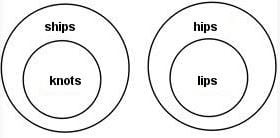Both Conclusions I and II follow

RRB JE ECE (CBT I) Mock Test- 4 - Question 42

In the following question, select the number which can be placed at the sign of question mark (?) from the given alternatives.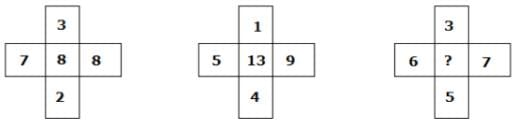Detailed Solution for RRB JE ECE (CBT I) Mock Test- 4 - Question 42

We have,

In fig (i) → 7*8 + 3*2 = 56+6 = 62 → 6+2 = 8

In fig (ii) → 5*9 + 1*4 = 45+4 = 49→ 4+9 = 13

Similarly,

In fig (iii) → 6*7 + 3*5 = 42+15= 57 → 5+7= 12

RRB JE ECE (CBT I) Mock Test- 4 - Question 43

In each of the questions below, one statement is given, followed by two conclusions which may or may not follow. From the options below, choose the one that reflects the correct choice of conclusion(s) that follows/follows.

National curriculums don't always work for rural and regional schools.

I. Curriculums should be tailor-made for rural and regional schools as per their requirements and specifications.

II. While formulating national curriculums, authorities don't always take the diversity of rural and regional schools into consideration.

Detailed Solution for RRB JE ECE (CBT I) Mock Test- 4 - Question 43

Both statements I and II are valid conclusions of the main statements. I- it talks about curriculums to be tailor-made since they don't always work for rural and regional schools as mentioned in the main statement. II talks about neglecting the diversity while formulating national curriculums which can be a reason for them not working.

RRB JE ECE (CBT I) Mock Test- 4 - Question 44

Each of the questions below consists of a question and two statements given below it. You have to decide whether the data provided in the statements are sufficient to answer the question.

Among M, P, K, J, T and W, who is lighter than only the heaviest?

I. P is heavier than M and T.

II. W is heavier than P but lighter than J who is not the heaviest.

Detailed Solution for RRB JE ECE (CBT I) Mock Test- 4 - Question 44 rom statement I, P > M, T

from statement II, J>W>P

From both the statements, J>W>P> M, T. Therefore K is the heaviest. So J is lighter than only the heaviest.

RRB JE ECE (CBT I) Mock Test- 4 - Question 45

If S=19 and SON=24 then SIT=?

Detailed Solution for RRB JE ECE (CBT I) Mock Test- 4 - Question 45 The average of the position values is taken

SIT= (19+9+20)/2 =24

RRB JE ECE (CBT I) Mock Test- 4 - Question 46

Select the suitable figure from the Answer figures that would replace the question mark (?).

Question Figure: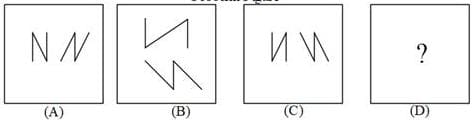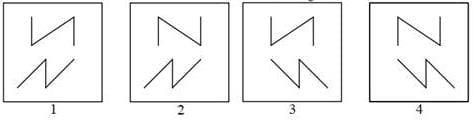Detailed Solution for RRB JE ECE (CBT I) Mock Test- 4 - Question 46

Option b is the correct answer.

Option a, c are incorrect as the object on top is wrongly drawn.

Option d is incorrect as the object at the bottom is wrongly drawn.

RRB JE ECE (CBT I) Mock Test- 4 - Question 47

Find out the odd term in the given series.

21,24, 81, 36, 6, 4

Detailed Solution for RRB JE ECE (CBT I) Mock Test- 4 - Question 47 Each term is a multiple of 3, except 4. Hence answer option is (3).
RRB JE ECE (CBT I) Mock Test- 4 - Question 48

In the following question, select the related word from the given alternatives.

Tuberculosis:Lungs ∷ Jaundice: ?

Detailed Solution for RRB JE ECE (CBT I) Mock Test- 4 - Question 48 Tuberculosis affects lungs and jaundice affects the liver.
RRB JE ECE (CBT I) Mock Test- 4 - Question 49

Z is older than only two people. T is younger to both W and V. Y is younger to neither Z nor T, who is older than both S and Z. U is younger to at least three persons, none of whom is S. Who among the given persons is older than as many persons as is younger to?

Detailed Solution for RRB JE ECE (CBT I) Mock Test- 4 - Question 49

Z is older than only two people.

We get,

___ < ___ < Z

Then,

T is younger to both W and V.

We get,

T < W, V

Since,

Y is younger to neither Z nor T, who is older than both S and Z.

We get,

___ < ___ < Z < T < W, V, Y

Only S and U are left and, we know,

U is younger to at least three persons, none of whom is S.

So, we get,

S < U < Z < T < W, V, Y

Hence, T is the one who is younger as well as elder to the same number of persons i.e. 3 persons.

RRB JE ECE (CBT I) Mock Test- 4 - Question 50

In the following question, select the related numbers from the given alternatives.

7834 : 499 ∷ 5271 : ?

Detailed Solution for RRB JE ECE (CBT I) Mock Test- 4 - Question 50

7*(8-1)= 49

3*(4-1) = 9

We get 499.

Similarly,

5*(2-1) = 5

7*(1-1) =0

We get 50.

RRB JE ECE (CBT I) Mock Test- 4 - Question 51

In each of the questions given below, there are two statements followed by an inference. Mark the correct option accordingly.

(a) Collection of frequent flyer miles is not useful at all.

(b) All the airlines have their respective frequent flyer programmes.

Inference: It's okay not to enrol under frequent flyer programmes of any airline.

Detailed Solution for RRB JE ECE (CBT I) Mock Test- 4 - Question 51 There can be other benefits of the frequent flyer programme of an airline apart from frequent flyer miles; hence, it wouldn't be completely correct to say the statement mentioned in inference; hence, (c) is the right answer. (If frequent flyer miles were the only benefit of a frequent flyer programme; in that case, the inference is definitely true).
RRB JE ECE (CBT I) Mock Test- 4 - Question 52

Identify the diagram that best represents the relationship among the given classes.

Children, naughty, energetic

Detailed Solution for RRB JE ECE (CBT I) Mock Test- 4 - Question 52 ome children are naughty, some children are energetic, some energetic may be naughty
RRB JE ECE (CBT I) Mock Test- 4 - Question 53

A series is given, with one term missing. Choose the correct alternative from the given ones that will complete the series.

EHF, ILJ, MPN, ?

Detailed Solution for RRB JE ECE (CBT I) Mock Test- 4 - Question 53

E---+4--- I ----+4--- M ----+4--- Q

H---+4--- L ----+4--- P ----+4--- T

F---+4--- J ----+4--- N ----+4--- R

RRB JE ECE (CBT I) Mock Test- 4 - Question 54

Which one set of letters when sequentially placed at the gaps in the given letter series shall complete it?

MNO_MMN_OO_MM_NNN_OOO

Detailed Solution for RRB JE ECE (CBT I) Mock Test- 4 - Question 54 Pattern is - MNOOMMNNOOOMMMNNNOOOO
RRB JE ECE (CBT I) Mock Test- 4 - Question 55

BANKER is coded as SFLOBC, how CLERK will be coded?

Detailed Solution for RRB JE ECE (CBT I) Mock Test- 4 - Question 55 Reverse the letters and then +1 to each letter.
RRB JE ECE (CBT I) Mock Test- 4 - Question 56

Anantapur district in Andhra Pradesh is famous for

RRB JE ECE (CBT I) Mock Test- 4 - Question 57

India-Korea business summit 2018 was held in:

RRB JE ECE (CBT I) Mock Test- 4 - Question 58

Highly specialized form of ag­riculture in which crops like coffee, tea and rubber are cul­tivated refer to:

RRB JE ECE (CBT I) Mock Test- 4 - Question 59

Wrestler Vinesh Phogat has become the first Indian women athlete to win a gold medal at the Asian Games 2018 held in Jakarta, Indonesia. She hails from:

RRB JE ECE (CBT I) Mock Test- 4 - Question 60

Which state Government has signed a Memorandum of Understanding (MoU) with the All India Football Federation (AIFF)?

RRB JE ECE (CBT I) Mock Test- 4 - Question 61

Tughlakabad, a ruined fort situated in Delhi was built by Ghiyas-ud-din Tughlaq, the founder of the Tughlaq dynasty of the Delhi Sultanate.

Detailed Solution for RRB JE ECE (CBT I) Mock Test- 4 - Question 61 Article 110 of the Constitution defines the “Money Bill”
RRB JE ECE (CBT I) Mock Test- 4 - Question 62

The 33rd ASEAN Summit is being chaired by whom?

Detailed Solution for RRB JE ECE (CBT I) Mock Test- 4 - Question 62 The summit is being chaired by Singapore’s Prime Minister Lee Hsien Loong.
RRB JE ECE (CBT I) Mock Test- 4 - Question 63

Which of the following was authored by Mulk Raj Anand?

Detailed Solution for RRB JE ECE (CBT I) Mock Test- 4 - Question 63

Mulk Raj Anand wrote the book “The Village” in the year 1939. Mulk Raj Anand December, 1905-28 September, 2004) was an Indian writer in English, notable for his depiction of the lives of the poorer castes in traditional Indian Society. He is one of the renowned authors of Inelo-Anglican fiction who together with R.K. Narayan, Ahmed Ali and Raja Rao, was one of the first India based writers in English to gain and International readership poetry essays, autobiographies and novels are included in his work on a wide range of subjects.

RRB JE ECE (CBT I) Mock Test- 4 - Question 64

Which is the second highest mountain in India?

Detailed Solution for RRB JE ECE (CBT I) Mock Test- 4 - Question 64 Nanda Devi is the second highest mountain in India.
RRB JE ECE (CBT I) Mock Test- 4 - Question 65

Who is the current Vice-Chairman of NITI Aayog?

RRB JE ECE (CBT I) Mock Test- 4 - Question 66

Which of the following is not a foodgrain?

RRB JE ECE (CBT I) Mock Test- 4 - Question 67

The sultans of which dynasty ruled for the largest time?

Detailed Solution for RRB JE ECE (CBT I) Mock Test- 4 - Question 67 The Tughlaq dynasty of North India was established in 1321 under Gazi Tughlaq i.e. Ghujasuddin Tughlaq and ended with the last ruler Nasiruddin Mahmud in 1412 A.D. One of the most controversial ruler of Delhi belongs to this dynasty Mohammed bin Tughlaq, who had

brought the idea of introducing token currency for the first time in India

RRB JE ECE (CBT I) Mock Test- 4 - Question 68

Who had saved the Delhi Sultanate from Chengiz Khan?

RRB JE ECE (CBT I) Mock Test- 4 - Question 69

Which of the following is located in Fatehpur Sikri?

RRB JE ECE (CBT I) Mock Test- 4 - Question 70

Which of the following cities was not built by Firoz Shah Tughlaq?

Detailed Solution for RRB JE ECE (CBT I) Mock Test- 4 - Question 70 Tughlaqabad, a ruined fort situated in Delhi was built by Ghiyas-ud-din Tughlaq, the founder of the Tughlaq dynasty of the Delhi Sultanate.
RRB JE ECE (CBT I) Mock Test- 4 - Question 71

The two elements that are frequently used for making transistors are ___ and ___.

Detailed Solution for RRB JE ECE (CBT I) Mock Test- 4 - Question 71 Silicon and Germanium : Transistors are made of semiconductor chemical elements, usually Silicon, which belongs to Group IV in the periodic table of elements. Germanium, another group-IV element, is used together with silicon in specialized transistors.
RRB JE ECE (CBT I) Mock Test- 4 - Question 72

The metal used in storage batteries —

RRB JE ECE (CBT I) Mock Test- 4 - Question 73

What is the orbital speed of a satellite rotating near the surface of earth?

Detailed Solution for RRB JE ECE (CBT I) Mock Test- 4 - Question 73 The orbital speed of a satellite rotating near the surface of earth is 7.9 km/sec
RRB JE ECE (CBT I) Mock Test- 4 - Question 74

Which of the following has the smallest atomic radius?

Detailed Solution for RRB JE ECE (CBT I) Mock Test- 4 - Question 74 The atomic size increases down the group.
RRB JE ECE (CBT I) Mock Test- 4 - Question 75

What should be the Mach number of a body for being hypersonic?

Detailed Solution for RRB JE ECE (CBT I) Mock Test- 4 - Question 75 The Mach number should be more than 5, i.e. more than 5 times the speed of sound
RRB JE ECE (CBT I) Mock Test- 4 - Question 76

Shearing stress that acts on a body affects its—

Detailed Solution for RRB JE ECE (CBT I) Mock Test- 4 - Question 76 A shearing stress that acts on a body affects its shape
RRB JE ECE (CBT I) Mock Test- 4 - Question 77

A light year is nearest to:

Detailed Solution for RRB JE ECE (CBT I) Mock Test- 4 - Question 77 ‎1 Light Year = 9.4607 × 1015 m almost equal to 1016 m
RRB JE ECE (CBT I) Mock Test- 4 - Question 78

Which of the following gases is lighter than air ?

Detailed Solution for RRB JE ECE (CBT I) Mock Test- 4 - Question 78 Ammonia: Anhydrous ammonia gas is lighter than air. However, in the presence of moisture (such as high relative humidity), the liquefied anhydrous ammonia gas forms vapors that are heavier than air. Methane, Hydrogen and helium Nitrogen are also lighter than air.
RRB JE ECE (CBT I) Mock Test- 4 - Question 79

The element required for Solar energy conversion is ____.

Detailed Solution for RRB JE ECE (CBT I) Mock Test- 4 - Question 79 Silicon is required for the conversion of solar energy.
RRB JE ECE (CBT I) Mock Test- 4 - Question 80

A moving body of mass 20 kg has 40 joules of kinetic energy. Calculate its speed?

RRB JE ECE (CBT I) Mock Test- 4 - Question 81

Ex situ conservation is carried out in

RRB JE ECE (CBT I) Mock Test- 4 - Question 82

The behaviour of a perfect gas, undergoing any change in the variables which control physical properties, is governed by

RRB JE ECE (CBT I) Mock Test- 4 - Question 83

Light Year is a unit to measure -

RRB JE ECE (CBT I) Mock Test- 4 - Question 84

Which of the following belongs to the 18th group of the Periodic Table?

RRB JE ECE (CBT I) Mock Test- 4 - Question 85

Who was the first person to describe plants?

RRB JE ECE (CBT I) Mock Test- 4 - Question 86

In screw jack, effort required to lift a load is given by:

RRB JE ECE (CBT I) Mock Test- 4 - Question 87

Which of the following is used for removing air bubbles from glass during its manufacture ?

RRB JE ECE (CBT I) Mock Test- 4 - Question 88

Deficiency of vitamin D causes —

RRB JE ECE (CBT I) Mock Test- 4 - Question 89

Which one of the following is the reason for small liquid drops assuming a spherical stage?

Detailed Solution for RRB JE ECE (CBT I) Mock Test- 4 - Question 89 The liquid tends to have minimum surface area due to surface tension. Small liquid drops from spherical shape because it wants to have less area due to the application of the surface tension.
RRB JE ECE (CBT I) Mock Test- 4 - Question 90

A plant used to treat bone fractures

RRB JE ECE (CBT I) Mock Test- 4 - Question 91

The most abundant metal in the earth’s crust is-

Detailed Solution for RRB JE ECE (CBT I) Mock Test- 4 - Question 91 Almost 99% of the minerals making up the Earth’s crust are made up of just eight elements.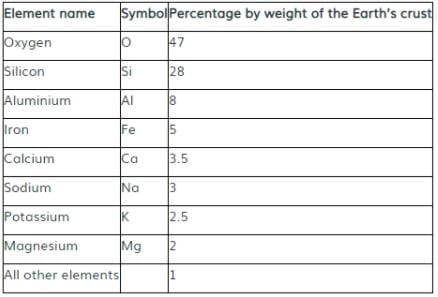RRB JE ECE (CBT I) Mock Test- 4 - Question 92

Kanha National Park is located in

RRB JE ECE (CBT I) Mock Test- 4 - Question 93

What changes will happen to a bowl of ice water kept at exactly zero degree Celsius?

RRB JE ECE (CBT I) Mock Test- 4 - Question 94

Hard Water contains which of the following?

Detailed Solution for RRB JE ECE (CBT I) Mock Test- 4 - Question 94 Hard water is generally found in areas where groundwater is in contact with limestone, chalk and dolomite and gets a higher amount of calcium and magnesium dissolved in it.
RRB JE ECE (CBT I) Mock Test- 4 - Question 95

A hydrogen balloon floats up because of:

RRB JE ECE (CBT I) Mock Test- 4 - Question 96

If an object is placed at between F and 2F in front of a Convex Lens, then the image formed will be

Detailed Solution for RRB JE ECE (CBT I) Mock Test- 4 - Question 96 The image formed will be beyond 2F, enlarged, real and inverted.
RRB JE ECE (CBT I) Mock Test- 4 - Question 97

Which one of the following denotes inertial mass?

Detailed Solution for RRB JE ECE (CBT I) Mock Test- 4 - Question 97 F/a. Inertial mass is a mass parameter giving the inertial resistance to acceleration of the body when responding to all types of force.

M = F/q

RRB JE ECE (CBT I) Mock Test- 4 - Question 98

A machine having an efficiency less than 50%, is known as:

RRB JE ECE (CBT I) Mock Test- 4 - Question 99

L.P.G. is a hydrocarbon consisting of a mixture of:

RRB JE ECE (CBT I) Mock Test- 4 - Question 100

In an atomic nucleus, neutrons and protons are held together by —

## RRB JE Mock Test Series for ECE

10 tests
 Use Code STAYHOME200 and get INR 200 additional OFF Use Coupon Code
Information about RRB JE ECE (CBT I) Mock Test- 4 Page
In this test you can find the Exam questions for RRB JE ECE (CBT I) Mock Test- 4 solved & explained in the simplest way possible. Besides giving Questions and answers for RRB JE ECE (CBT I) Mock Test- 4, EduRev gives you an ample number of Online tests for practice

10 tests